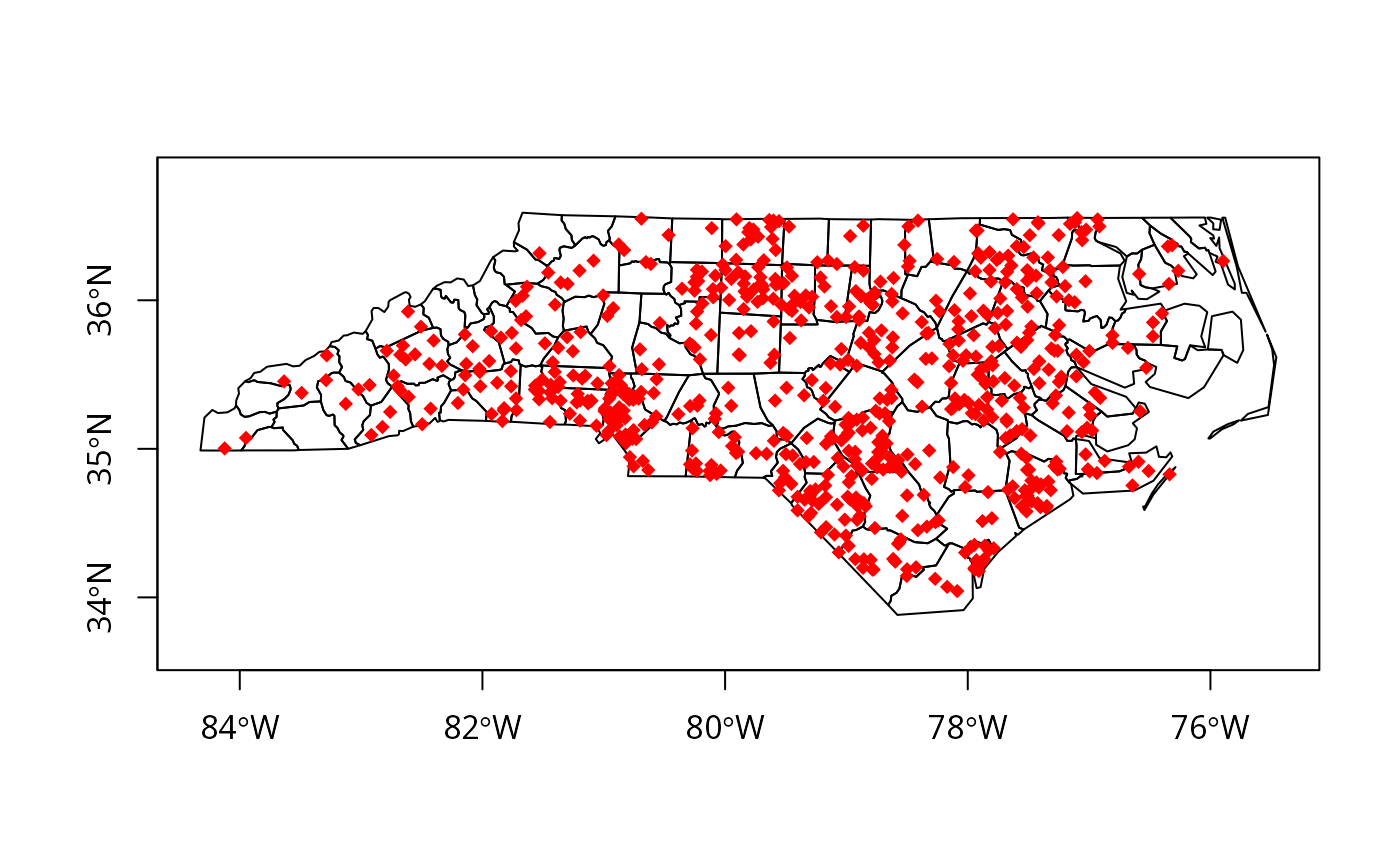Make point coordinates for a dot density map

dotsInPolys(pl, x, f = "random", offset, compatible = FALSE)

## Arguments

pl an object of class SpatialPolygons or SpatialPolygonsDataFrame integer vector of counts of same length as pl for dots type of sampling used to place points in polygons, either "random" or "regular" for regular sampling only: the offset (position) of the regular grid; if not set, c(0.5,0.5), that is the returned grid is not random what to return, if TRUE a a list of matrices of point coordinates, one matrix for each member of pl, if false a SpatialPointsDataFrame with polygon ID values

## Details

With f="random", the dots are placed in the polygon at random, f="regular" - in a grid pattern (number of dots not guaranteed to be the same as the count). When the polygon is made up of more than one part, the dots will be placed in proportion to the relative areas of the clockwise rings (anticlockwise are taken as holes). From maptools release 0.5-2, correction is made for holes in the placing of the dots, but depends on hole values being correctly set, which they often are not.

## Value

If compatible=TRUE, the function returns a list of matrices of point coordinates, one matrix for each member of pl. If x[i] is zero, the list element is NULL, and can be tested when plotting - see the examples. If compatible=FALSE (default), it returns a SpatialPointsDataFrame with polygon ID values as the only column in the data slot.

## Note

Waller and Gotway (2004) Applied Spatial Statistics for Public Health Data (Wiley, Hoboken, NJ) explicitly warn that care is needed in plotting and interpreting dot density maps (pp. 81-83)

## Author

Roger Bivand Roger.Bivand@nhh.no

spsample

## Examples

nc_SP <- readShapePoly(system.file("shapes/sids.shp", package="maptools"),
proj4string=CRS("+proj=longlat  +ellps=clrk66"))
#> Warning: readShapePoly is deprecated; use rgdal::readOGR or sf::st_read#> Warning: Discarded datum Unknown based on Clarke 1866 ellipsoid in Proj4 definitionif (FALSE) {
pls <- slot(nc_SP, "polygons")
pls_new <- lapply(pls, checkPolygonsHoles)
nc_SP <- SpatialPolygonsDataFrame(SpatialPolygons(pls_new,
proj4string=CRS(proj4string(nc_SP))), data=as(nc_SP, "data.frame"))
}
try1 <- dotsInPolys(nc_SP, as.integer(nc_SP$SID74)) plot(nc_SP, axes=TRUE) plot(try1, add=TRUE, pch=18, col="red")try2 <- dotsInPolys(nc_SP, as.integer(nc_SP$SID74), f="regular")
plot(nc_SP, axes=TRUE)